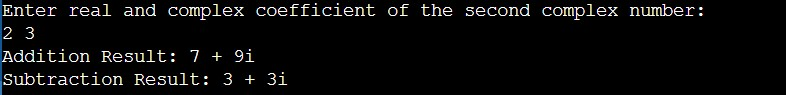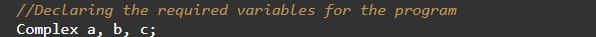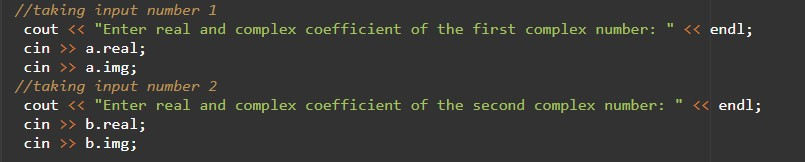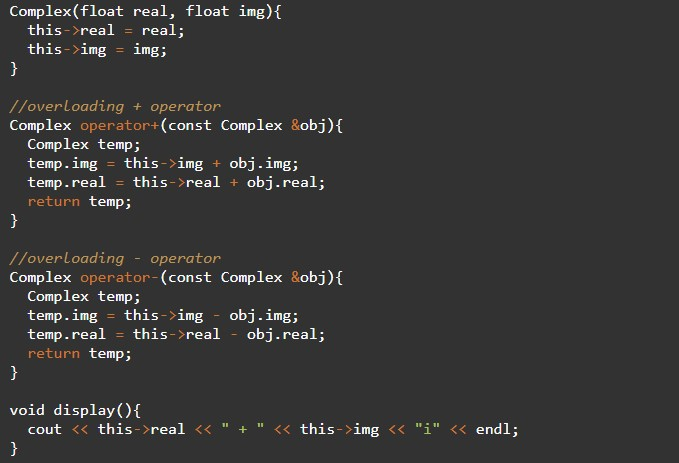In this tutorial you will learn about the C++ Program to Subtract Complex Number Using Operator Overloading and its application with practical example.

In this tutorial, we will learn to create a C++ program that will Subtract Complex Number Using Operator Overloading in C++ programming.

## Prerequisites.

Before starting with this tutorial we assume that you are best aware of the following C++ programming topics:

• Operators in C++ Programming.
• Basic Input and Output function in C++ Programming.
• Basic C++ programming.
• While loop in C++ programming.

## What is a Complex Number?

A number is said to be a complex number if it is in the form of x+yi, where x and y are real numbers, and i is an indeterminate satisfying the condition i2 = −1.

For example, 2 + 3i

## Algorithm:-

In this program, we will subtract a Complex Number Using Operator Overloading. First, we will take the numbers input from the user and second, we will solve that number using operator overloading.

## Output:-In the above program, we have first initialized the required variable.• a = it will hold the complex value.
• b = it will hold the complex value.
• c = it will hold the complex value.
• temp = it will hold the complex value.
• real = it will hold the float value.
• img = it will hold the integer value.

Taking the input integer number and exponent from the user.Calculating the complex numbers.Printing the output numbers.In this tutorial we have learn about the C++ Program to Subtract Complex Number Using Operator Overloading and its application with practical example. I hope you will like this tutorial.